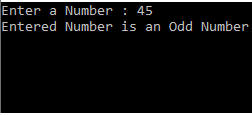# Even Odd Program in C# using IF ELSE

In the blog, You will learn How to Find Odd Event Numbers using the If Else Statement in C#. Write a program to check whether a user input number is even or odd in CSharp.

## Problem Description

The C# Program checks if a given user input integer is Odd or Even.

## Problem Solution

In the program, if a user input number is divisible by 2 with the remainder 0 then the number is an Even number. If the number is not divisible or remainder by 2 then that number will be an Odd number.

## % Operator in C# :

% operator computes the remainder after dividing one number by another. x % y will return the remainder after dividing x by y. In the given example

```using System;namespace FindAndSolve{
public class Program  {  public static void Main(string[] args)  {   Console.WriteLine(7 % 3);   Console.WriteLine(6 % 3);
Console.WriteLine(16 % 4);   Console.WriteLine(8 % -3);   Console.WriteLine(-10 % 8);   Console.WriteLine(-16 % -9);        }    }
}```

#### Output

1

0

0

2

-2

-7

The above example is dealing with only integers but we can also use % with floating-point numbers. For floating-point numbers, the result will be a float value.

## Source Code Example

`using System;// namespace declarationnamespace HelloWordInCsharp{    // declaration class here    public class FindAndSolve    {        // This is Main Method         public static void Main(string[] args)        {            int userInput;            Console.Write("Enter a Number : ");            userInput = int.Parse(Console.ReadLine());            if (userInput % 2 == 0)            {                Console.Write("Entered Number is an Even Number");            }            else            {                Console.Write("Entered Number is an Odd Number");            }        }    }}`

#### Output## Odd Event Number Program Explanation

In this given above example, you are reading the number using ‘userInput’ integer variable. If a conditional statement is used to check the number is even and odd. For an even number, the modulus of the value "userInput" variable by 2 is equal to zero, if the condition is true then print the statement as an even number.

Otherwise, if the condition is false then execute the else statement, for odd number the modulus of the value of ‘userInput’ variable by 2 is not equal to zero, if the condition is true then execute the statement and print the statement as an odd number.